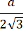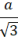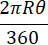# Area of Rectangle, Square, Triangle, circle and more

## AREA

I. (i) Area of Rectangle =  length ✕ width

(ii) Perimeter of Rectangle = 2 ( length + width )

II. (i) Area of Square = side ✕ side   = 1/2 ✕ diagonal ✕ diagonal

(ii) Diagonal of Square = ( √2 ✕ side )

III. Area of the four walls of the room = 2 ( length + width ) ✕ height

IV. (i) Area of triangle = 1/2  base ✕ height

(ii) Area of triangle =    where  s = 1/2 (a+b+c) and a,b,c side of triangle.

(iii) Area of equilateral triangle = √3/4 ✕ side ✕ side
(iv) The radius of the inner circle of the equilateral triangle with 'a' side =(v) The radius of the circumcircle of equilateral triangle with 'a' side =V. (i) Area of a parallelogram = base ✕ height
= a ✕ h

(ii) Area of rhombus = 1/2 ✕ (Product of diagonals)
= 1/2 ✕ (a✕ b)

(iii) Half of the diagonals of a rhombus and one side of the rhombus form a right-angled triangle whose hypotenuse is the side of the quadrilateral.

VI. Area of trapezium = 1/2 ✕ sum of parallel side ✕ distance between them
= 1/2 ✕ (a+b) ✕ h

VII. (i) Area of circle = πR2
(ii) Circumference of circle = 2πR
(iii) Area of semi-circle = 1/2 ✕ πR2
(iv) Perimeter of semi-circle = (πR + 2R)

(v) Arc length =(vi) Area of the circle segment AOB = 1/2 ✕ (arc AB) ✕ R =